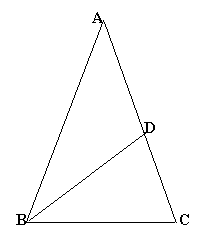#### You may also like### Gold Again

Without using a calculator, computer or tables find the exact values of cos36cos72 and also cos36 - cos72.### Pythagorean Golden Means

Show that the arithmetic mean, geometric mean and harmonic mean of a and b can be the lengths of the sides of a right-angles triangle if and only if a = bx^3, where x is the Golden Ratio.### Golden Powers

You add 1 to the golden ratio to get its square. How do you find higher powers?

# Golden Triangle

##### Age 16 to 18Challenge LevelThe three triangles $ABC$, $CBD$ and $ABD$ are all isosceles. Find the angles in the triangles.

The sides $AB$ and $BC$ have lengths $p$ and $q$ respectively. Prove that the ratio $p/q$ is equal to the golden ratio $\frac{1}{2} (\sqrt{5}\ +1)$.

and find the ratio $q/p$.

The area of triangle $ABC$ is 2 square units. Find the areas of $CBD$ and $ABD$ exactly (i.e. find the areas in the form $a + b \sqrt{5}$

where $a$ and $b$ are rational numbers)